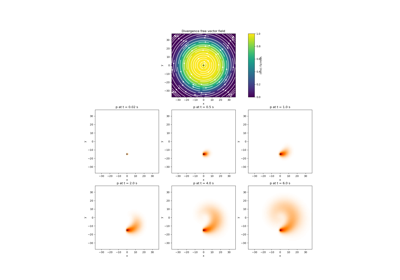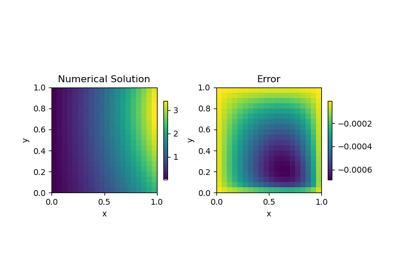# discretize.mixins.InterfaceVTK#class discretize.mixins.InterfaceVTK[source]#

VTK interface for discretize meshes.

Class enabling straight forward conversion between discretize meshes and their corresponding VTK or PyVista data objects. Since InterfaceVTK is inherritted by the BaseMesh class, this functionality can be called directly from any discretize mesh! Currently this functionality is implemented for CurvilinearMesh, TreeMesh and discretize.TensorMesh classes; not implemented for CylindricalMesh.

It should be noted that if your mesh is defined on a reference frame that is not the traditional <X,Y,Z> system with vectors of $$(1,0,0)$$, $$(0,1,0)$$, and $$(0,0,1)$$, then the mesh in VTK will be rotated so that it is plotted on the traditional reference frame; see examples below.

Examples

The following are examples which use the VTK interface to convert discretize meshes to VTK data objects and write to VTK formatted files. In the first example example, a tensor mesh whose axes lie on the traditional reference frame is converted to a pyvista.RectilinearGrid object.

>>> import discretize
>>> import numpy as np
>>> h1 = np.linspace(.1, .5, 3)
>>> h2 = np.linspace(.1, .5, 5)
>>> h3 = np.linspace(.1, .8, 3)
>>> mesh = discretize.TensorMesh([h1, h2, h3])


Get a VTK data object

>>> dataset = mesh.to_vtk()


Save this mesh to a VTK file

>>> mesh.write_vtk('sample_mesh')


Here, the reference frame of the mesh is rotated. In this case, conversion to VTK produces a pyvista.StructuredGrid object.

>>> axis_u = (1,-1,0)
>>> axis_v = (-1,-1,0)
>>> axis_w = (0,0,1)
>>> mesh.orientation = np.array([
...    axis_u,
...    axis_v,
...    axis_w
... ])


Yield the rotated vtkStructuredGrid

>>> dataset_r = mesh.to_vtk()


or write it out to a VTK format

>>> mesh.write_vtk('sample_rotated')


The two above code snippets produced a pyvista.RectilinearGrid and a pyvista.StructuredGrid respecitvely. To demonstarte the difference, we have plotted the two datasets next to each other where the first mesh is in green and its data axes are parrallel to the traditional cartesian reference frame. The second, rotated mesh is shown in red and its data axes are rotated from the traditional Cartesian reference frame as specified by the orientation property.

>>> import pyvista
>>> pyvista.set_plot_theme('document')

>>> p = pyvista.BackgroundPlotter()
>>> p.show_grid()
>>> p.screenshot('vtk-rotated-example.png')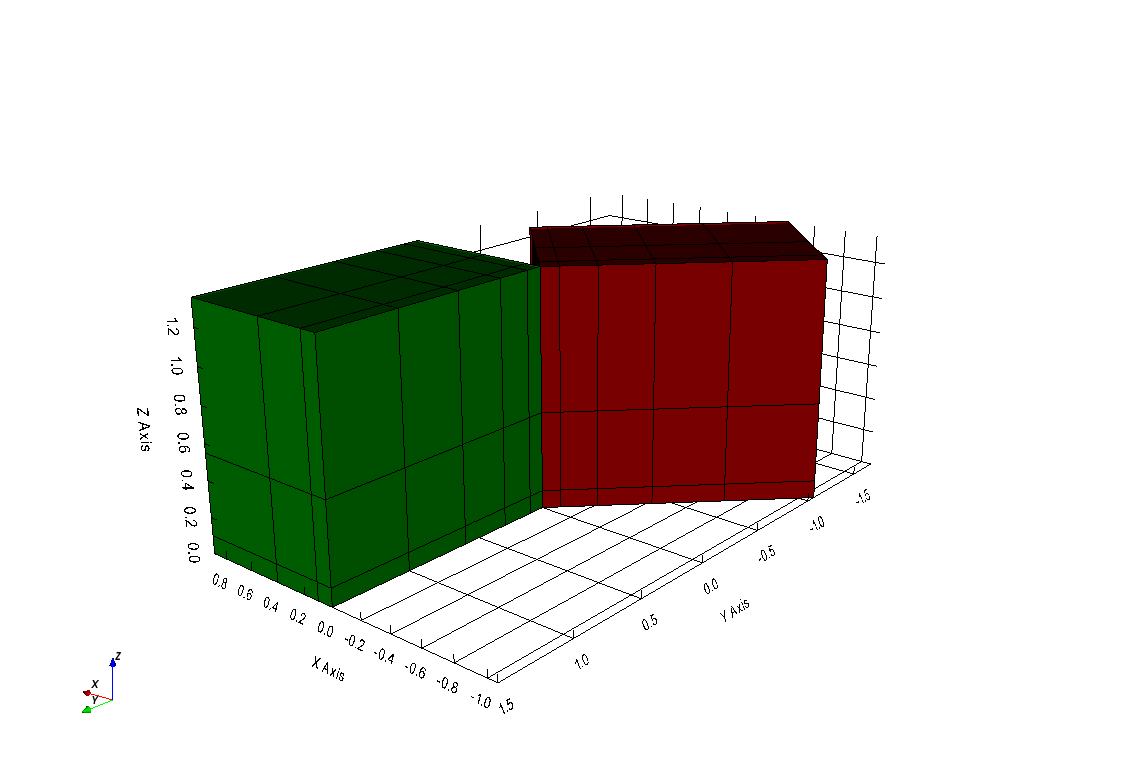Methods

 toVTK([models]) Convert mesh (and models) to corresponding VTK or PyVista data object. to_vtk([models]) Convert mesh (and models) to corresponding VTK or PyVista data object. writeVTK(file_name[, models, directory]) Convert mesh (and models) to corresponding VTK or PyVista data object then writes to file. write_vtk(file_name[, models, directory]) Convert mesh (and models) to corresponding VTK or PyVista data object then writes to file.

## Galleries and Tutorials using discretize.mixins.InterfaceVTK#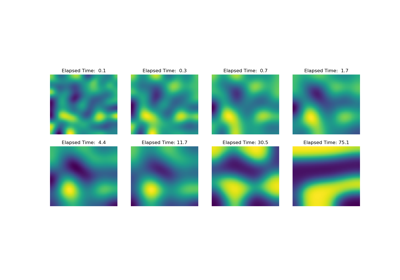Operators: Cahn Hilliard

Operators: Cahn Hilliard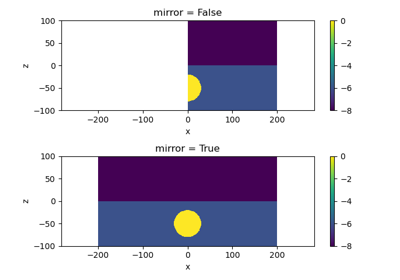Plot Mirrored Cylindrically Symmetric Model

Plot Mirrored Cylindrically Symmetric Model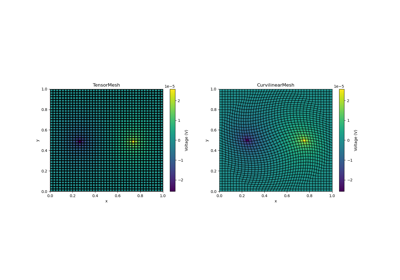Basic Forward 2D DC Resistivity

Basic Forward 2D DC ResistivityBasic: PlotImage

Basic: PlotImage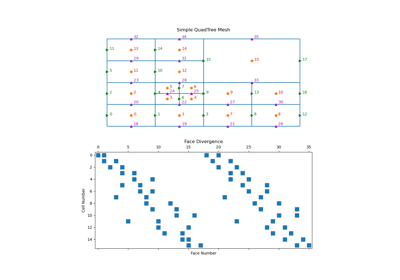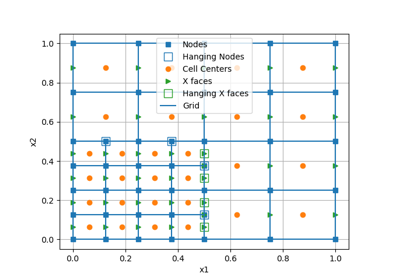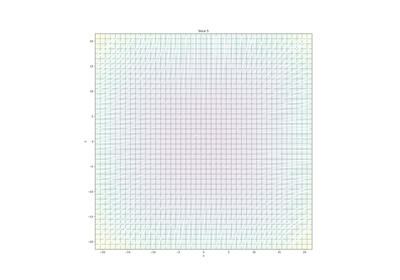Plotting: Streamline thickness

Plotting: Streamline thickness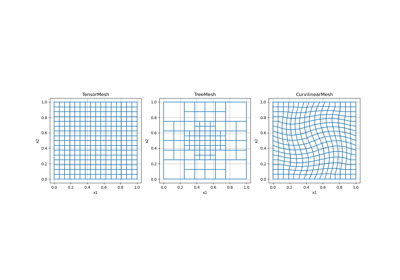Overview of Mesh Types

Overview of Mesh Types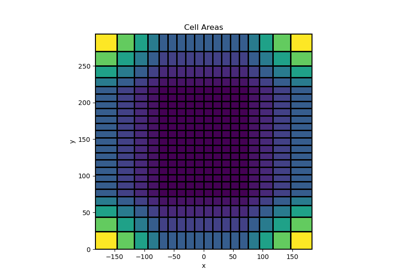Tensor meshes

Tensor meshes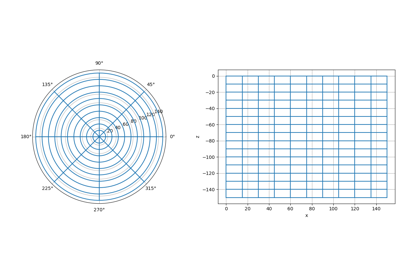Cylindrical meshes

Cylindrical meshes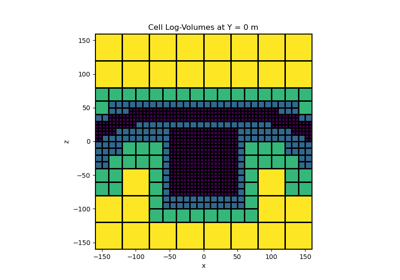Tree Meshes

Tree Meshes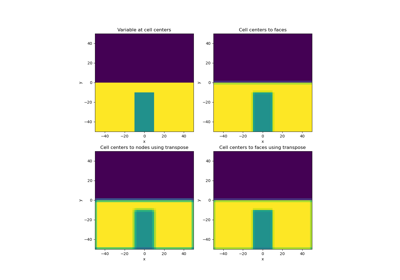Averaging Matricies

Averaging Matricies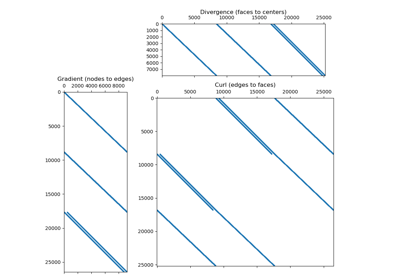Differential Operators

Differential Operators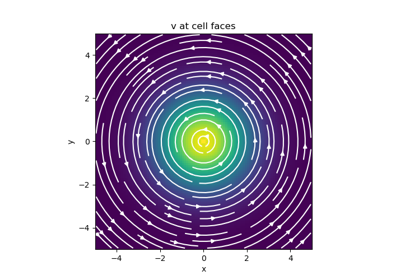Basic Inner Products

Basic Inner Products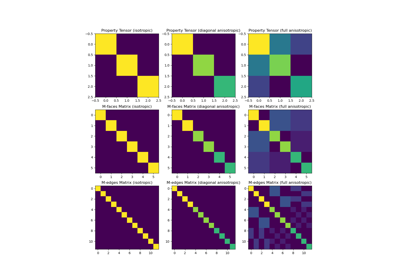Constitutive Relations

Constitutive Relations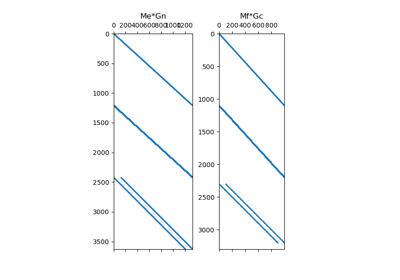Differential Operators

Differential Operators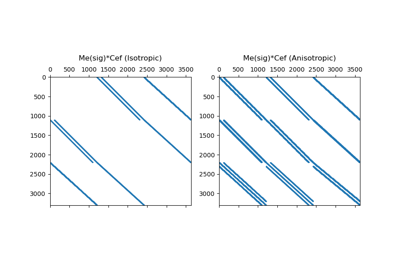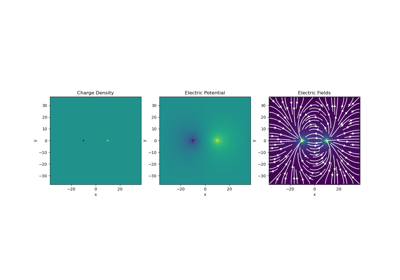Gauss’ Law of Electrostatics

Gauss' Law of Electrostatics